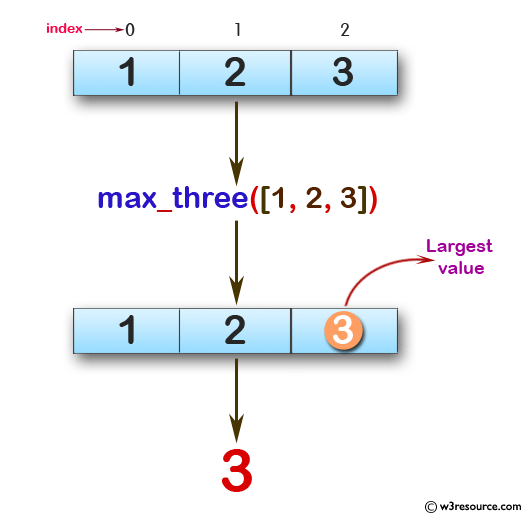﻿ Swift Array Programming Exercise: Find the largest value from the first, last, and middle values in a given array of integers and length will be at least 1 - w3resource# Swift Array Programming Exercises: Find the largest value from the first, last, and middle values in a given array of integers and length will be at least 1

## Swift Array Programming: Exercise-21 with Solution

Write a Swift program to find the largest value from the first, last, and middle values in a given array of integers and length will be at least 1.

Pictorial Presentation:Sample Solution:

Swift Code:

`````` func max_three(_ a: [Int]) -> Int {
guard a.count % 2 == 1 else {
return 0
}

let middle_index = a.count / 2

if a.first! > a.last! && a.first! > a[middle_index] {
return a.first!
} else if a.last! > a.first! && a.last! > a[middle_index] {
return a.last!
} else {
return a[middle_index]
}
}

print(max_three([1, 2, 3]))
print(max_three([-1, -5, -7]))
print(max_three([0, 1, 9]))
```
```

Sample Output:

```3
-1
9
```

Swift Programming Code Editor:

Improve this sample solution and post your code through Disqus

What is the difficulty level of this exercise?

﻿